# Graphing Quadratic Functions General Form It is assumed

• Slides: 15
Download presentationGraphing Quadratic Functions – General Form • It is assumed that you have already viewed the previous three slide shows titled Graphing Quadratic Functions – Concept Graphing Quadratic Functions – Standard Form Graphing Quadratic Functions – Converting General Form To Standard Form • The next three pages are summaries from those shows, and are important to understanding this module. Table of ContentsStandard Form of a Quadratic Function General Form of a Quadratic Function Table of Contents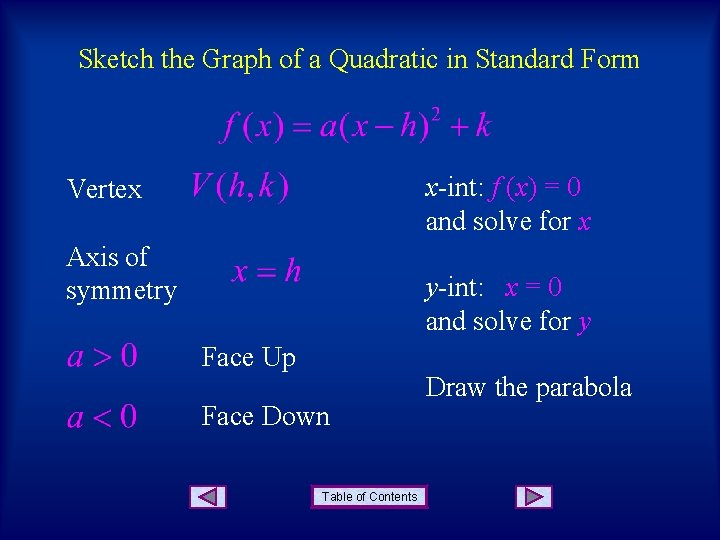Sketch the Graph of a Quadratic in Standard Form x-int: f (x) = 0 and solve for x Vertex Axis of symmetry y-int: x = 0 and solve for y Face Up Face Down Table of Contents Draw the parabola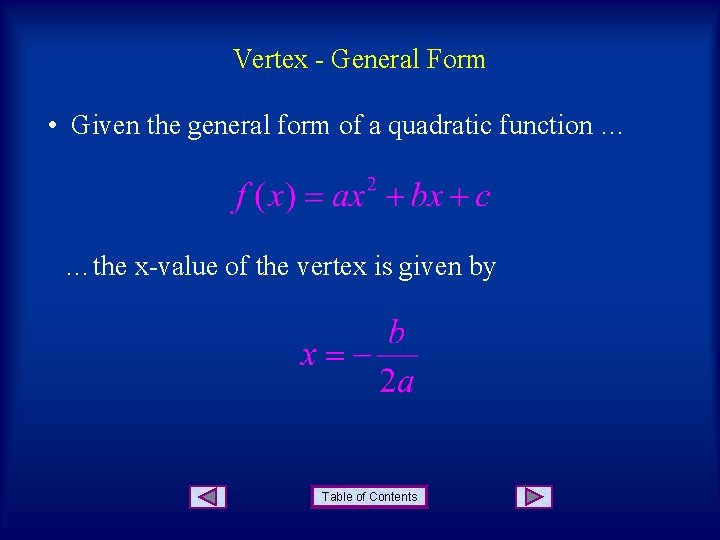Vertex - General Form • Given the general form of a quadratic function … …the x-value of the vertex is given by Table of Contents• There are two other helpful concepts for graphing a quadratic function that is in general form. • Concept 1: We already know that the x-value of the vertex is given by … To find the y-value of the vertex, just plug the x-value into the function … Table of Contents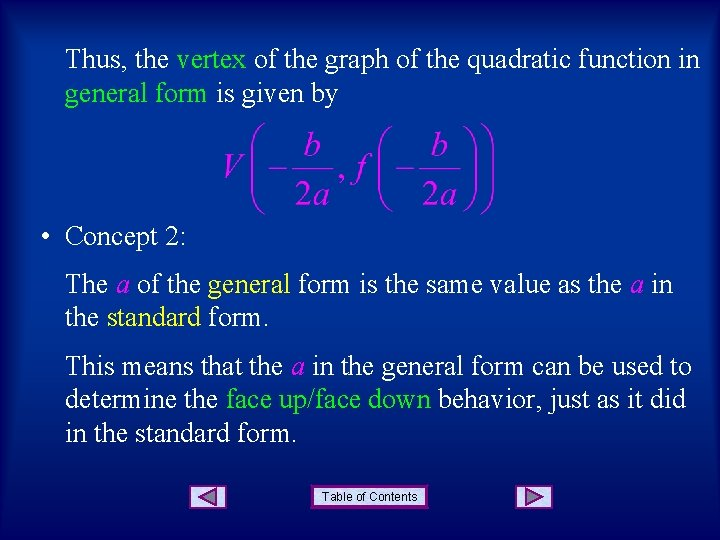Thus, the vertex of the graph of the quadratic function in general form is given by • Concept 2: The a of the general form is the same value as the a in the standard form. This means that the a in the general form can be used to determine the face up/face down behavior, just as it did in the standard form. Table of Contents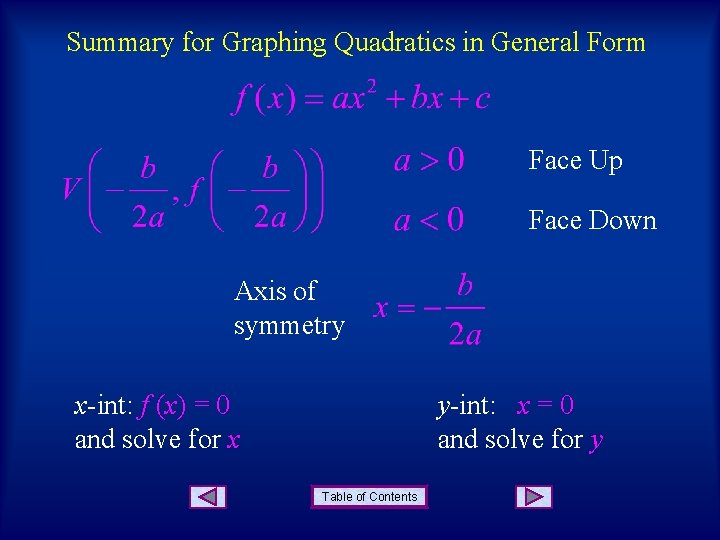Summary for Graphing Quadratics in General Form Face Up Face Down Axis of symmetry x-int: f (x) = 0 and solve for x y-int: x = 0 and solve for y Table of Contents• Example: Sketch the graph of the given quadratic function. Face down Vertex: Table of Contents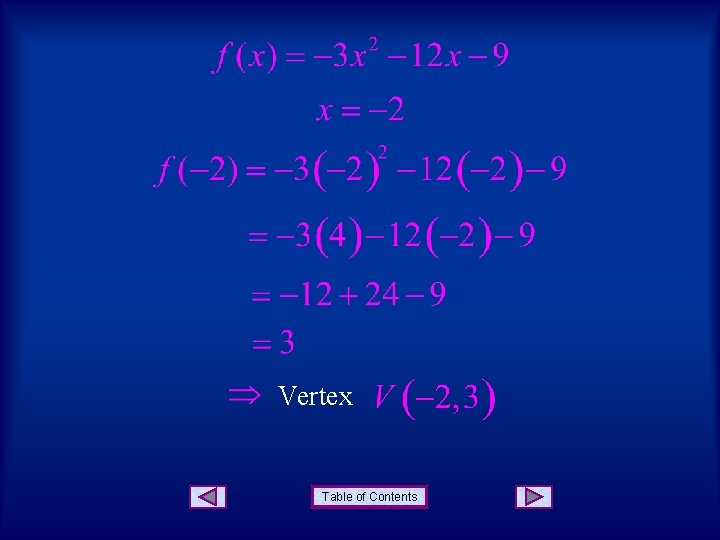Vertex Table of ContentsAxis of Symmetry: x = x-value of vertex Table of Contentsx-intercepts Table of Contents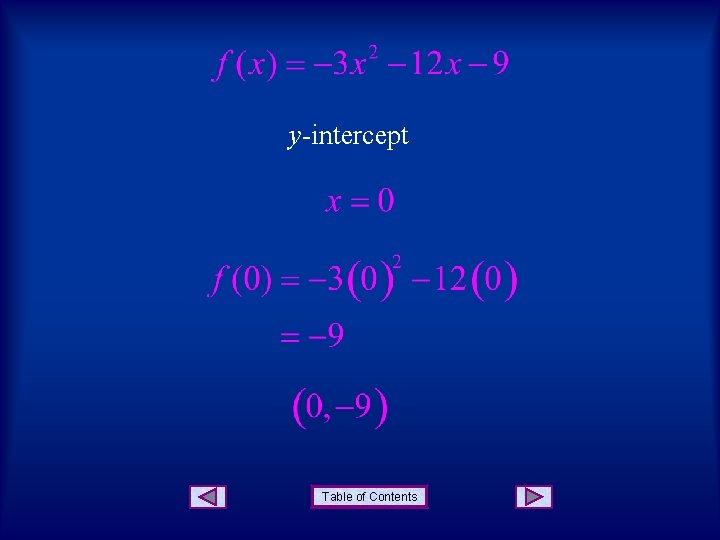y-intercept Table of ContentsSummary: Function: Face down x-intercepts y-intercept Axis: Table of Contents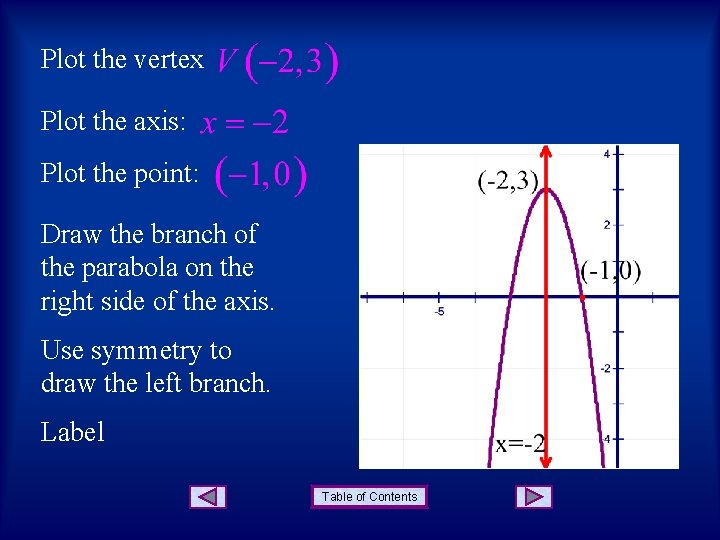Plot the vertex Plot the axis: Plot the point: Draw the branch of the parabola on the right side of the axis. Use symmetry to draw the left branch. Label Table of ContentsTable of Contents